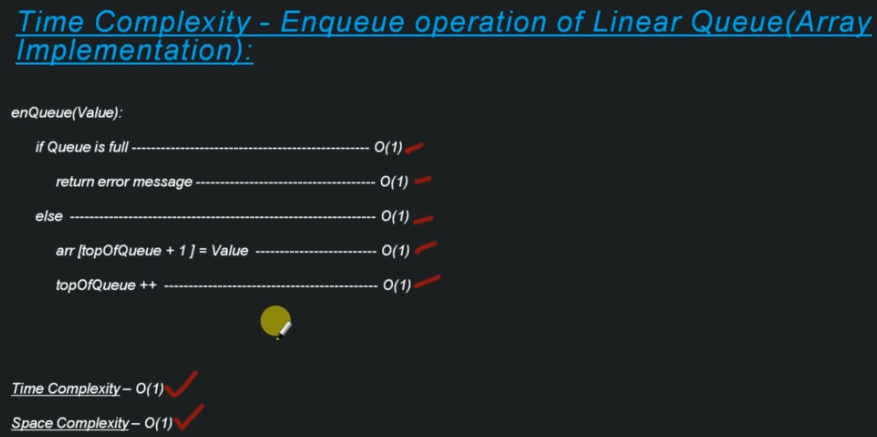# Data Structures & Algorithms part-7 ( Queue )

A queue is a linear structure which follows a particular order in which the operations are performed. The order is First In First Out.

# Linear queue (Array implementation)first insert 10 then 20, then 30 for dequeue operation first dequeue 10 then 20 then 30

# Circular queue

In linear queue when we dequeue value from queue, dequeue value from the start. so at the time of dequeue we can’t use this front space. If we utilize space then we should implement Circular queue.

Circular Queue is a linear data structure in which the operations are performed based on FIFO (First In First Out) principle and the last position is connected back to the first position to make a circle. It is also called ‘Ring Buffer’. In a normal Queue, we can insert elements until queue becomes full.

If we are trying to dequeue an element and there is no element in the queue then we simply return error message.

# Linear queue ( Linked list implementation )

In dequeue we have two operation over here.

One if the queue is empty then simply return error message.

then if there is at least one element in the queue then we can perform dequeue successfully .